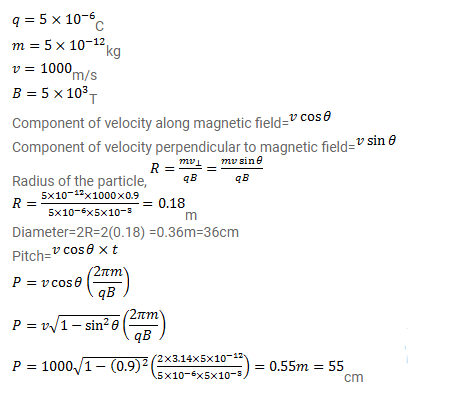# A particle having a charge of 0.5mc and a mass of

Question:

A particle having a charge of $5.0 \mu \mathrm{C}$ and a mass of $5.0 \times 10^{-12} \mathrm{~kg}$ is projected with a speed of $1.0 \mathrm{~km} / \mathrm{s}$ in a magnetic field of magnitude $5.0 \mathrm{mT}$. The angle between the magnetic field and the velocity is $\sin ^{-1}(0.90)$. Show that the path of the particle will be a helix. Find the diameter of the helix and its pitch.

Solution: